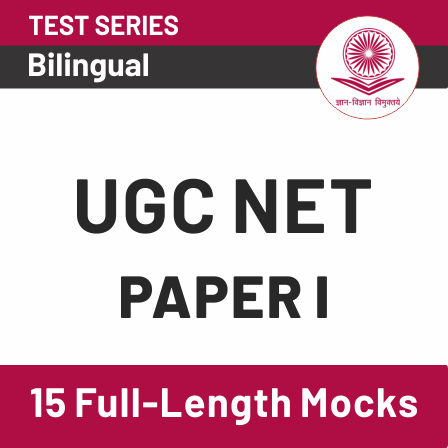# UGC NET Previous Year Question December 2019 For Logical & Mathematical ReasoningLogical & Mathematical Reasoning are  important section in UGC NET Exam 2020.  UGC NET is an entrance exam for the selection of deserving candidates for the posts of Assistant Professor and Junior Research Fellowship (JRF).

Get free study Material for UGC NET 2020

## Highlights :

Exam Name UGC NET
Subject Reasoning (including Maths), Logical Reasoning, Data Interpretation
Total Questions 5 (for each)
Total Marks 10 (for each)
Marking For right answer – 2 mark
Negative Marking No
Question Pattern Objective type
Language Bilingual
Mathematical Reasoning and Aptitude
• Types of reasoning.
• Number series, Letter series, Codes and Relationships.
• Mathematical Aptitude (Fraction, Time & Distance, Ratio, Proportion and Percentage, Profit and Loss, Interest and Discounting, Averages etc.).
##### Logical Reasoning
• Understanding the structure of arguments: argument forms, structure of categorical propositions, Mood and Figure, Formal and Informal fallacies, Uses of language, Connotations and denotations of terms, Classical square of opposition.
• Evaluating and distinguishing deductive and inductive reasoning.
• Venn diagram: Simple and multiple use for establishing validity of arguments.
• Indian Logic: Means of knowledge.
##### Data Interpretation
• Sources, acquisition and classification of Data.
• Quantitative and Qualitative Data.
• Graphical representation (Bar-chart, Histograms, Pie-chart, Table-chart and Line-chart) and mapping of Data.
• Data Interpretation.
• Data and Governance.

Here we are going to provide you quizzes related to UGC NET syllabus which contains mainly flowing topics i.e. Reasoning (including Maths), Logical Reasoning, Data Interpretation

### Today Quiz : UGC NET Previous Yr Quiz Dec 2019

Q1. What is the missing term in the series given below?

2, 4, 6, 12, 10, 36, 14, ?,  18, 324 . . . . . . . . . . . .

(a) 50

(b) 72

(c) 108

(d) 18

Q2. Apoorav is son of Madhu. Vinod is brother of Madhu’s husband. How is Apoorav related to Vinod?

(a) Uncle

(b) Brother

(c) Nephew

(d) Cousin

Q3. In a certain code “ENGLAND” is written as “ULSNYLV”. Using the same code. “IRELAND” will be written as

(a) HQUNYLV

(b) QHUNYLV

(c) QUHNYLV

(d) HUQNYLV

Q4. The ratio of two numbers a and b is 3 : 7. After adding 9 to each number, the ratio becomes 9 : 17. The numbers a and b are:

(a) (6, 14)

(b) (9, 21)

(c) (15, 35)

(d) (18, 42)

Q5. The next term in the series AT,  EQ, IN, OK . . . . . . . . . . . .  is

(a) UH

(b) UP

(c) US

(d) UU

Q6. “All students cleared their examination” and “Few students did not clear their examination”.  This is an instance of __________________________ .

(b) Superaltern

(c) Subaltern

(d) Contraries

Q7. Product of two consecutive integers is divisible by 2. Therefore 4 × 5 (=20) is divisible by 2. This is an example of which type of Reasoning?

(a) Abductive

(b) Informal

(c) Inductive

(d) Deductive

Q8. The distinction between laukika and alaukika is made with reference to which one of the following pramānas?

(a) Anumāna (Inference)

(b) Upamāna (Comparison)

(c) Pratyaksa (Perception)

(d) S’abda (Verbal testimony)

Directions (9 – 13) :  The following table gives the percentage of marks obtained by five students in five subjects. Maximum marks in each subject are given in the parenthesis below the subject name.

 Student Percentage (%) Marks obtained Maths (100) Chemistry (100) Physics (100) English (100) Physical Education (100) A 90 80 95 80 90 B 80 75 90 70 80 C 100 95 95 85 95 D 75 70 60 65 60 E 60 50 65 50 70

Q9. The aggregate of marks obtained by E is:

(a) 252.5

(b) 251.5

(c) 353.5

(d) 352.5

Q10. What is the difference between aggregate marks obtained by A and C?

(a) 40.5

(b) 41.5

(c) 42.5

(d) 48.5

Q11. In which subject, the students have obtained lowest average (%) percentage of marks?

(a) Chemistry

(b) Physics

(c) English

(d) Physical Education

Q12. The aggregate percentage of marks obtained by C are :

(a) 92.20%

(b) 94.17%

(c) 95.21%

(d) 91.17%

Q13. What is the average percentage of marks obtained by the students in Chemistry?

(a) 74%

(b) 79%

(c) 80%

(d) 81%

How to Crack UGC NET While Working?

Solutions

S1. Ans.(c)

Sol.S2. Ans.(c)

Sol.S3. Ans.(b)

Sol.S4. Ans.(d)

Sol. Acc. to QuestionsS5. Ans.(a)

Sol.S6. Ans.(a)

S7. Ans.(d)

Sol. Deductive

S8. Ans.(c)

Sol. Pratyaksha (Perception)

UGC NET Salary 2020

S9. Ans.(d)

Sol. Marks of E in Maths, Chemistry, Physics, English and Physical Education respectively = 60, 75, 97.5, 50, 70,

So, Aggregate marks = 60 + 75 + 97.5 + 50 + 70

⟹ 352.5

S10. Ans.(c)

Sol. Aggregate marks obtained by A ⟹  90 + 120 + 142.5 + 80 + 90 = 522.5

Aggregate marks obtained by C ⟹ 100 + 142.5 + 142.5 + 85 + 95 = 565

Difference = 565 – 522.5 ⟹ 42.5

S11. Ans.(c)

Sol.S12. Ans.(b)

Sol.S13. Ans.(a)

Sol.Get free study Material for UGC NET 2020

For More Details :